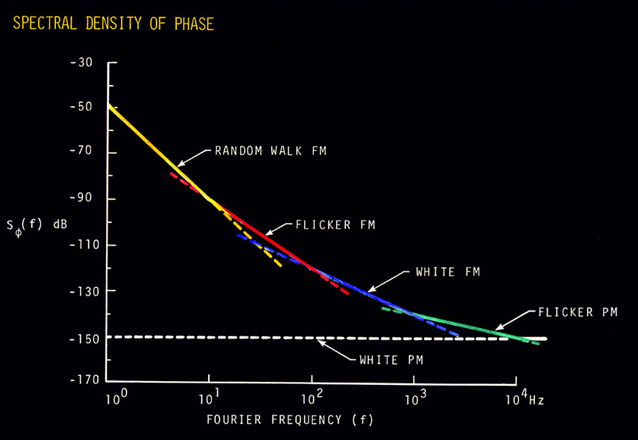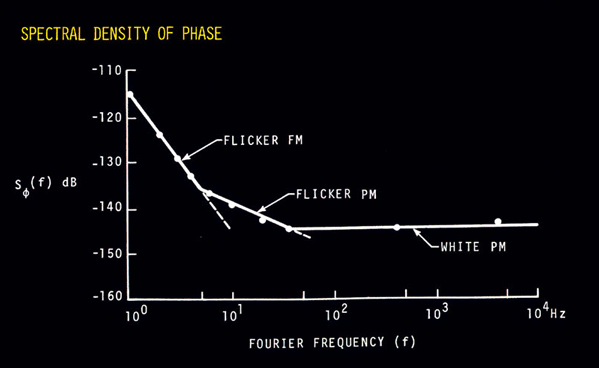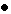IX. POWER-LAW NOISE PROCESSES Power-law noise processes are models of precision oscillator noise that produce a particular slope on a spectral density plot. We often classify these noise processes into one of five categories. For plots of Sf (f), they are: 1.Random walk FM (random walk of frequency), Sf plot goes down as 1/f4. 2.Flicker FM (flicker of frequency), Sf plot goes down as 1/f3. 3.White FM (white of frequency), Sf plot goes down as 1/f2. 4.Flicker PM (flicker of phase), Sf plot goes down as 1/f. 5.White PM (white of phase), Sf plot is flat. Power law noise processes are characterized by their functional dependence on Fourier frequency. Equation 8.7 relates Sf (f) to Sy(f), the spectral density of frequency fluctuations. Translation of Sy(f) to time-domain data s y(t ) for the five model noise processes is covered later in section XI.Figure 9.1: Power-law noise is indicated by a particular slope in the phase-noise measurement. The spectral density plot of a typical oscillator's output usually is a combination of different power-law noise processes. It is very useful and meaningful to categorize the noise processes. The first job in evaluating a spectral density plot is to determine which type of noise exists for a particular range of Fourier frequencies. It is possible to have all five noise processes being generated from a single oscillator, but, in general, only two or three noise processes are dominant. Figure 9.1 is a graph of Sf (f) showing the five noise processes on a log-log scale. Figure 9.2 shows the spectral density of phase fluctuations for a typical high-quality oscillator.Figure 9.2: Different power-law noises have different causes in an oscillators output signal. Main PageTable of ContentsGo to section: Summary and IntroductionIIIIIIIVVVIVIIVIIIIXXXIXIIConclusionReferences